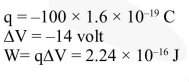# Two points P and Q are maintained at the potential of`
Question:

Two points $\mathrm{P}$ and $\mathrm{Q}$ are maintained at the potential of $10 \mathrm{~V}$ and $-4 \mathrm{~V}$, respectively. The work done in moving 100 electrons from $P$ to $Q$ is :-

1. $-2.24 \times 10^{-16} \mathrm{~J}$

2. $2.24 \times 10^{-16} \mathrm{~J}$

3. $-9.60 \times 10^{-17} \mathrm{~J}$

4. $9.60 \times 10^{-17} \mathrm{~J}$

Correct Option: , 2

Solution: